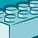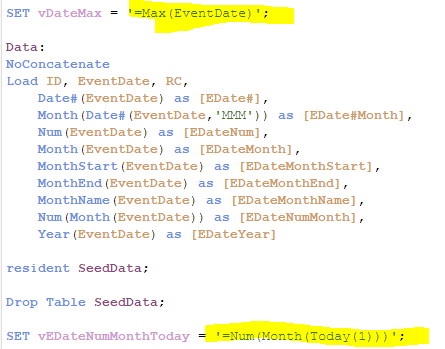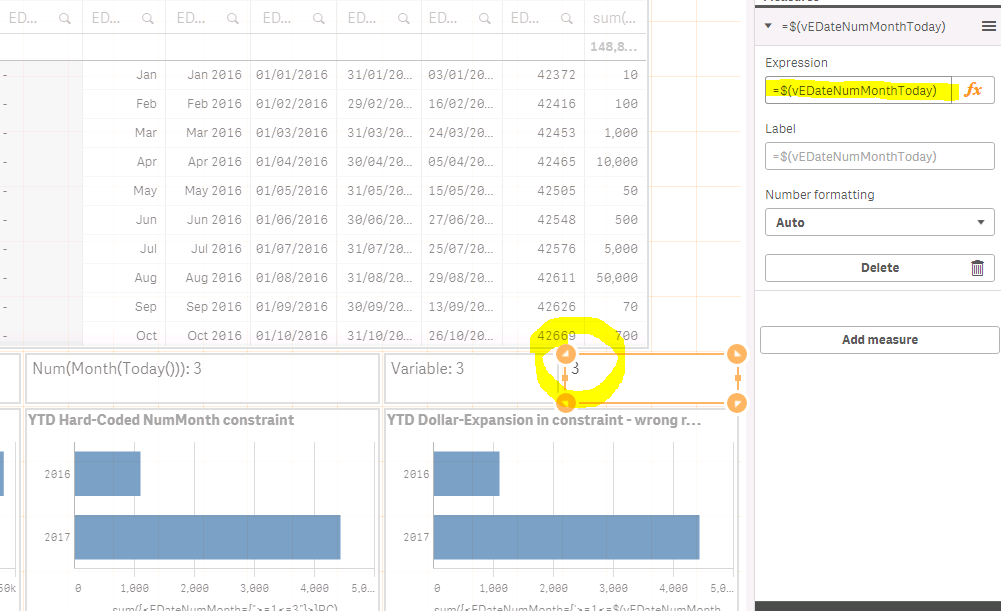# App Development

Discussion board where members can learn more about Qlik Sense App Development and Usage.

Announcements
Welcome to Qlik Community! Check out our new navigation! FIND OUT MORE
cancel
Showing results for
Did you mean:Contributor III

## Seeking correct syntax when using variable as constraint in set analysis

Dear Community

I'm using Qlik Sense 3.1 SR4 here; I'm aiming for something of a YTD calculation, based on the Year up to the point the data is loaded and it's being plotted in a stacked bar chart in comparison with previous years.

I have EventDate values in the data and from this I'm deriving EDateNumMonth from Num(Month(EventDate)), giving a number value for Month in each data row. Meanwhile, a control variable, vEDateNumMonthToday is set in the load script; Let's say we're in March, so 3 then.

Now in Qlikview (v11) I used an expression logic that ran like this:

sum({<FieldNumMonth={'<=\$(vTheCurrentMonth)'}>}RCounter).

This worked great and provided a YTD total for each year present in the data.

I'm trying to employ the same approach in our QlikSense rendition, but it seems something is skewed.

If I hard code the limitation, as:

sum({<EDateNumMonth={'<=3'}>}RC)

or  sum({<EDateNumMonth={'>=1<=3'}>}RC)

everything is fine - all adds up as it should.

As soon as I add a dollar expansion to the set analysis:

sum({<EDateNumMonth={'<=\$(vEDateNumMonthToday)'}>}RC) - the columns disappear, or

sum({<EDateNumMonth={'>=1<=\$(vEDateNumMonthToday)'}>}RC) - the columns display the total for the whole year!

Uuh?

Am I missing something here?

@MichaelTarallo, or other community colleagues; Any constructive suggestions would be most welcome.

Regards

Graham

1 Solution

Accepted SolutionsContributor III

Hi!

You should put an equal sign so that the variable calculatescurrently it is comparing it with a string Num(Month(Today(1)))

I tried changing your variable in the script asNow it computes3 RepliesContributor III

Hi!

You should put an equal sign so that the variable calculatescurrently it is comparing it with a string Num(Month(Today(1)))

I tried changing your variable in the script asNow it computesMVP

Or even a dollar sign expansion within the dollar sign expansion can work also

Sum({<EDateNumMonth={'>=1<=\$(=\$(vEDateNumMonthToday))'}>}RC)Contributor III
Author

Hey, Thank you for that insight. More attention to LET and SET would probably have been useful when I wrote the script.Tags
Community Browser